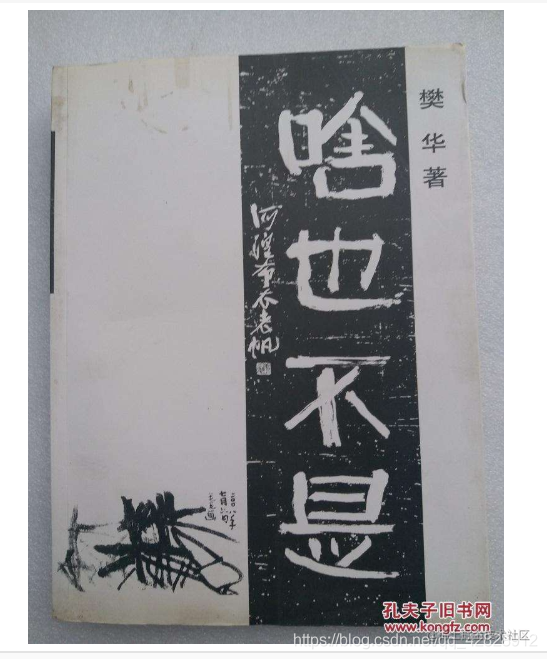# 为什么面试老是问HashMap# HashMap的底层实现原理

## 定义的常量及其使用

``````public class ConcurrentHashMap<K,V> extends AbstractMap<K,V>
implements ConcurrentMap<K,V>, Serializable {

private static final long serialVersionUID = 362498820763181265L;
static final int DEFAULT_INITIAL_CAPACITY = 1 << 4; //默认初始大小
static final int MAXIMUM_CAPACITY = 1 << 30;//最大容量
static final float DEFAULT_LOAD_FACTOR = 0.75f;//装载因子
static final int TREEIFY_THRESHOLD = 8;//链表最大长度
static final int UNTREEIFY_THRESHOLD = 6;//红黑树最小长度
static final int MIN_TREEIFY_CAPACITY = 64;//最小键值对数量

``````public abstract class AbstractMap<K,V> implements Map<K,V> {

``````这咋这么一大堆，听我慢慢道来

``````DEFAULT_INITIAL_CAPACITY 是默认初始大小 16
MAXIMUM_CAPACITY   是最大容量  1073741824
TREEIFY_THRESHOLD 是链表的最大长度  当链表长度超过8就会转换成红黑树
UNTREEIFY_THRESHOLD 是红黑树最小长度 当树长度小于6就会转换为链表
MIN_TREEIFY_CAPACITY  是最小键值对数量  当键值对超过64时才会转换

`````` public HashMap() {
}

`````` public HashMap(int initialCapacity, float loadFactor) {
if (initialCapacity < 0)
throw new IllegalArgumentException("Illegal initial capacity: " +
initialCapacity);
if (initialCapacity > MAXIMUM_CAPACITY)
initialCapacity = MAXIMUM_CAPACITY;
throw new IllegalArgumentException("Illegal load factor: " +
this.threshold = tableSizeFor(initialCapacity);
}

`````` static class Node<K,V> implements Map.Entry<K,V> {
final int hash;//对象的hash值
final K key;//键
V value;//值
Node<K,V> next;//指向下一个元素

## put（）方法详解

`````` public V put(K key, V value) {
return putVal(hash(key), key, value, false, true);
}

final V putVal(int hash, K key, V value, boolean onlyIfAbsent,
boolean evict) {
Node<K,V>[] tab; Node<K,V> p; int n, i;
if ((tab = table) == null || (n = tab.length) == 0)
n = (tab = resize()).length;
if ((p = tab[i = (n - 1) & hash]) == null)
tab[i] = newNode(hash, key, value, null);
else {
Node<K,V> e; K k;
if (p.hash == hash &&
((k = p.key) == key || (key != null && key.equals(k))))
e = p;
else if (p instanceof TreeNode)
e = ((TreeNode<K,V>)p).putTreeVal(this, tab, hash, key, value);
else {
for (int binCount = 0; ; ++binCount) {
if ((e = p.next) == null) {
p.next = newNode(hash, key, value, null);
if (binCount >= TREEIFY_THRESHOLD - 1) // -1 for 1st
treeifyBin(tab, hash);
break;
}
if (e.hash == hash &&
((k = e.key) == key || (key != null && key.equals(k))))
break;
p = e;
}
}
if (e != null) { // existing mapping for key
V oldValue = e.value;
if (!onlyIfAbsent || oldValue == null)
e.value = value;
afterNodeAccess(e);
return oldValue;
}
}
++modCount;
if (++size > threshold)
resize();
afterNodeInsertion(evict);
return null;
}

`````` static final int hash(Object key) {
int h;
return (key == null) ? 0 : (h = key.hashCode()) ^ (h >>> 16);
}

``````哈希函数 ，也叫散列值优化函数,也叫扰动函数

## 扩容机制

`````` ++modCount;
if (++size > threshold)
resize();
afterNodeInsertion(evict);
return null;

## 小经验分享

`````` final V putVal(int hash, K key, V value, boolean onlyIfAbsent,
boolean evict) {
Node<K,V>[] tab; Node<K,V> p; int n, i;
if ((tab = table) == null || (n = tab.length) == 0)
n = (tab = resize()).length;
if ((p = tab[i = (n - 1) & hash]) == null)//就是这里
tab[i] = newNode(hash, key, value, null);

## 最后给你们推荐一本书吧